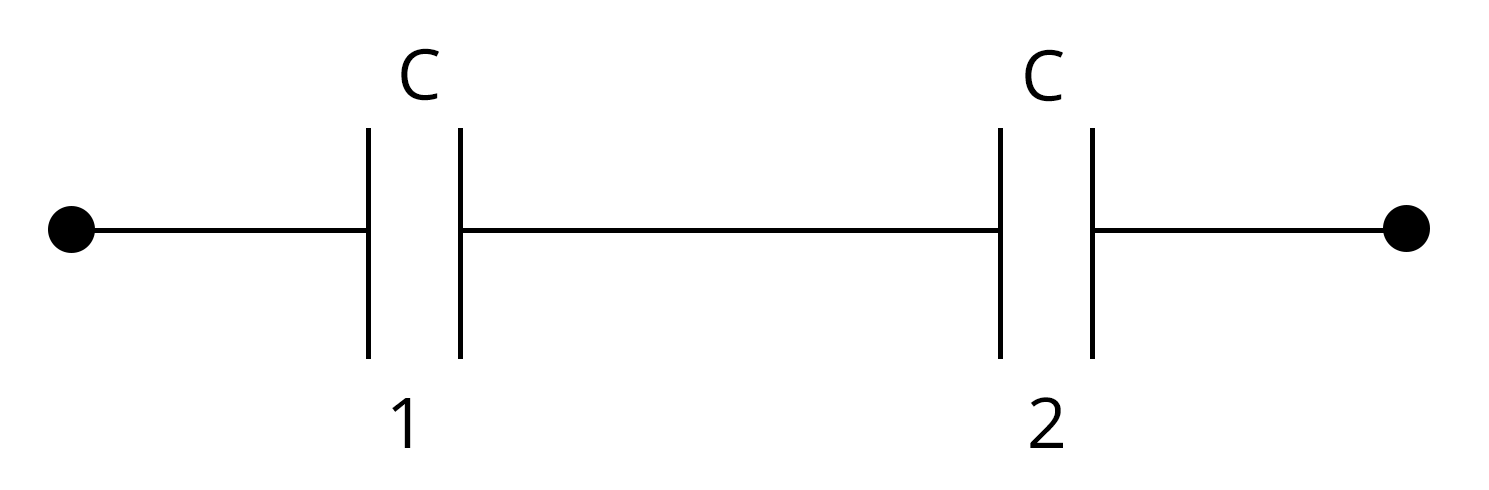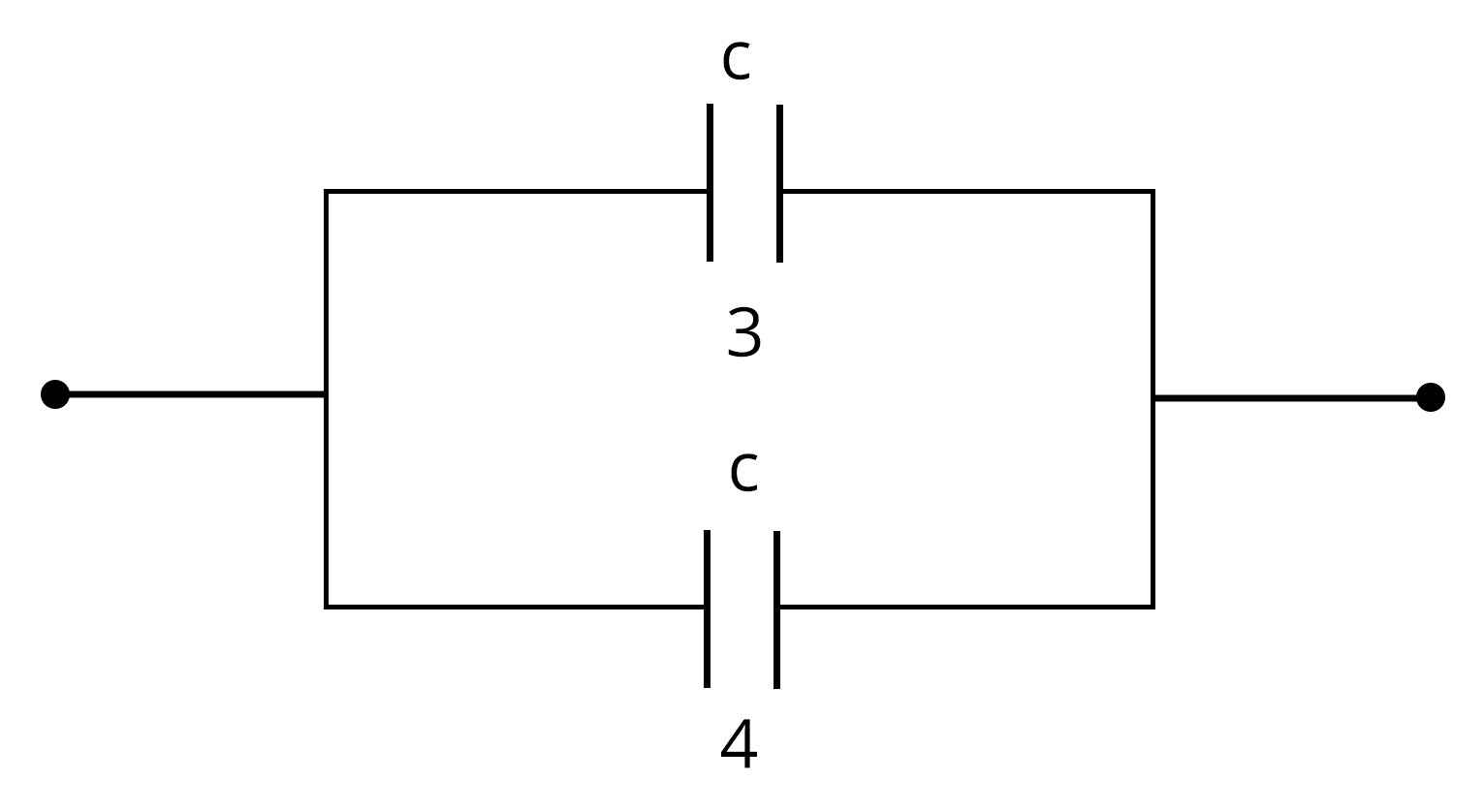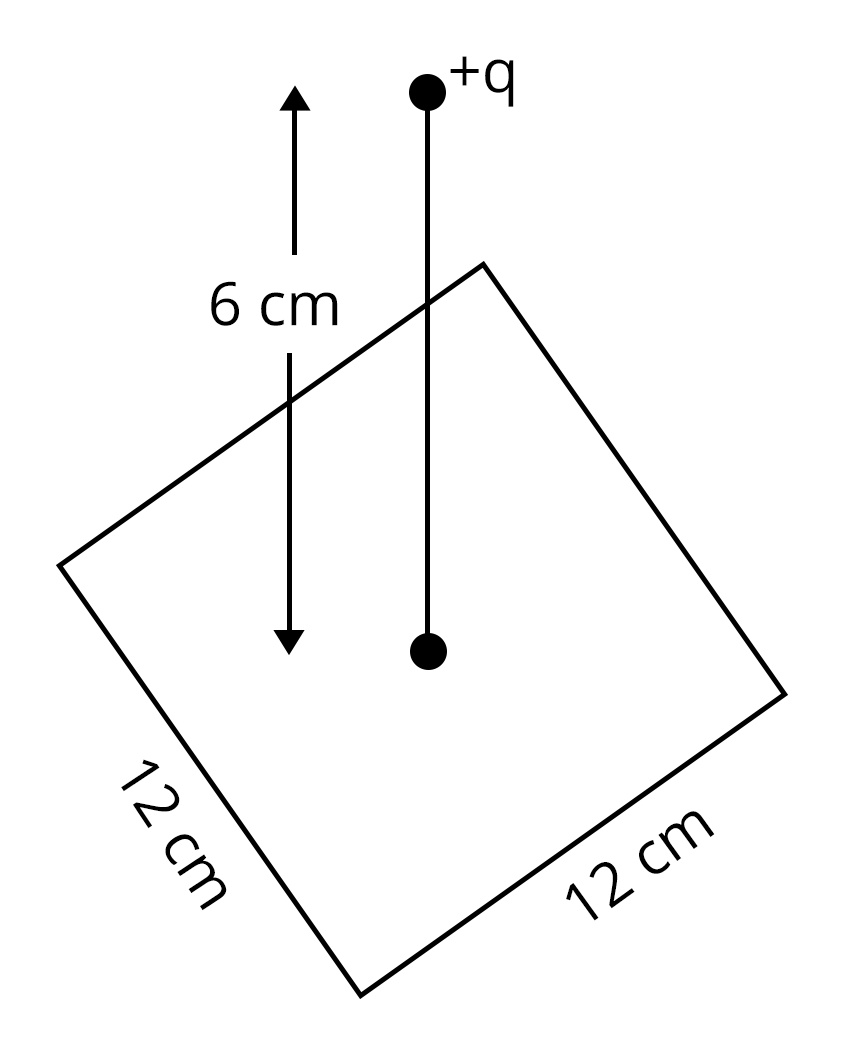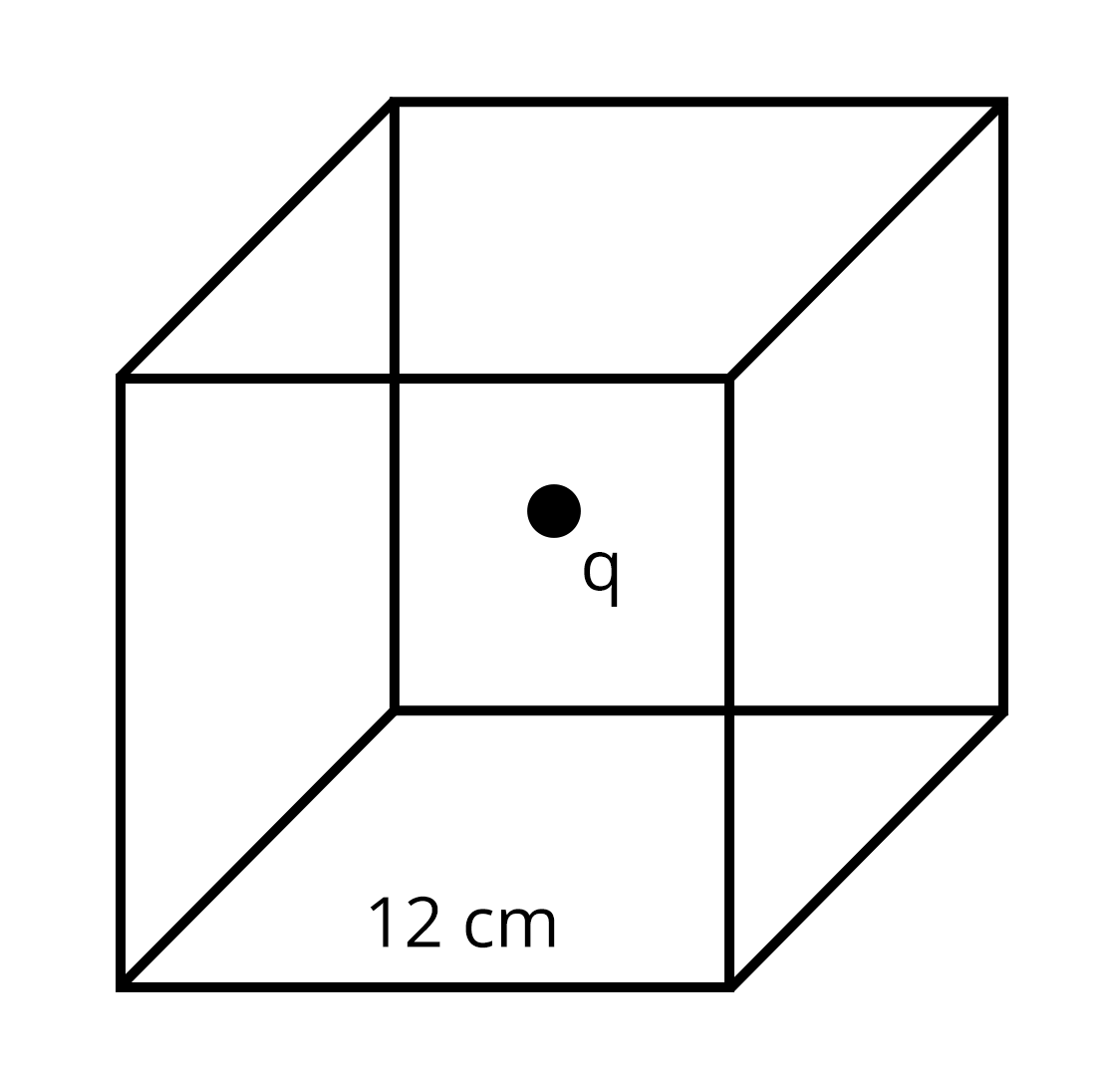Courses
Courses for Kids
Free study material
Offline Centres
More

# Electrostatics Chapter - Physics JEE MainLast updated date: 04th Dec 2023
Total views: 23.4k
Views today: 0.23k## Concepts of Electrostatics for JEE Main Physics

Electrostatics is a fundamental topic that spans concepts introduced in both Class 8 and Class 12. In Class 8, students are initiated into the basics of electrostatics, grasping the concept of electric charge and the fundamental law governing charges. Progressing to Class 12, Electrostatics delves deeper, exploring Coulomb's Law, electric field, and potential. Electrostatics in Class 12 students further delve into Gauss's law, capacitance, and the equilibrium of conductors and insulators in electrostatics. A comprehensive understanding of electrostatics is imperative for tackling advanced JEE Main problems related to electromagnetism and lays the groundwork for more intricate physics concepts.

The Electrostatics chapter commences by elucidating electrostatics, electric charge, and Coulomb’s law. It progresses to addressing problems related to Coulomb's law, electric potential, and electric field due to point charges and various charge distributions. Examples illustrating electrostatic effects in daily life are also examined. The chapter explores the torque and potential energy of an electric dipole, applying Gauss's law to calculate electric flux. JEE often features questions on capacitor types, alongside problems involving series and parallel combinations. Additionally, the impact of a dielectric medium in a capacitor is discussed. This section defines electrostatic force, outlines essential JEE Main and JEE Advanced formulas for electrostatics, and offers solved examples.

## JEE Main Physics Chapters 2024

### Important Topics of Electrostatics Chapter

• Coulomb’s Law.

• Electric field and electric lines of force.

• Electric field due to continuous charge distribution.

• Electric dipole and dipole moment.

• Gauss Law.

• Electric potential and Equipotential surface.

• Electric potential due to the various charge distributions.

• Capacitance of a capacitor.

• Grouping of Capacitance.

### What is Electrostatic?

Electrostatics is the study of electric charges at rest. It involves the interaction between charged particles and the forces and fields they create. Coulomb's law is a fundamental principle in electrostatics that describes the force between two-point charges.

### JEE Main Electrostatics Solved Examples

1. The electric potential in a region is given by V=(6x-8xy2-8y+6yz-4x2) volt. Then the electric field acting on a point charge of 2C placed at the origin will be …

Ans: Given,

The electric potential in a region , V=(6x-8xy2-8y+6yz-4x2) volt

The electric field in the region can be obtained from the electric potential using the formula

$\vec E=\dfrac{\partial V}{\partial x} \hat i+\dfrac{\partial V}{\partial y} \hat j+\dfrac{\partial V}{\partial z} \hat k$

$\vec E=(6-8y^2-8x) \hat i+(-16xy-8+6z) \hat j+6y \hat k$...(1)

To find the electric field at origin, put x=0, y=0 and z=0 in equation (1)

$\vec E=6 \hat i+-8 \hat j+0 \hat k$

$|\vec E|=\sqrt{6^2+(-8)^2}$

$|\vec E|=10~N/C$

The force acting on the charge q due to electric E is given by

$F=qE$

$F=2C\times 10~N/C=20~N$

Key point: Electric field at a point can be obtained by the differentiation of electric potential with respect to distance.

2. A parallel plate capacitor with oil between plates (dielectric constant of oil k=2) has a capacitance C. If the oil is removed, then the capacitance of the capacitor becomes…

Ans: Let A be the area of the capacitor and d be the distance between the plates of the capacitor.

The formula for the  capacitance of the parallel plate capacitor with oil as a dielectric is given  by,

$C=\dfrac{kA\epsilon_0}{d}$...(1)

The capacitance of the parallel plate capacitor when oil is removed is

$C'=\dfrac{A\epsilon_0}{d}$...(2)

Divide equation (2) by (1) to obtain the new capacitance C’ when oil is removed in terms of initial capacitance C.

$\dfrac{C'}{C}=\dfrac{\left(\dfrac{A\epsilon_0}{d}\right)}{\left(\dfrac{kA\epsilon_0}{d}\right)}$

$\dfrac{C'}{C}=\dfrac{1}{k}$

$C'=\dfrac{C}{k}$

When the oil is removed from the parallel plate capacitor, its capacitance is reduced by a factor of k.

Key point:  The capacitance of a parallel plate capacitor depends on the dielectric medium and the dimensions of the capacitor. It does not depend on the charge and potential difference across the capacitor.

### Previous Year Questions from JEE Paper

1. Two equal capacitors are first connected in series and then in parallel. The ratio of equivalent capacitances in the two cases will be (JEE 2021)

Sol:

Let the capacitances of each capacitor be C.

In the series connection of two capacitors, the equivalent capacitances is given by$\dfrac{1}{C_{eq}}=\dfrac{1}{C_1}+\dfrac{1}{C_2}$

$\dfrac{1}{C_{12}}=\dfrac{1}{C}+\dfrac{1}{C}$

$C_{12}=\dfrac{C}{2}$...(1)

For the capacitors in parallel connection, the equivalent capacitance is given by$C_{eq}=C_{3}+C_{4}$

$C_{34}=C+C$

$C_{34}=2C$...(2)

The ratio of  the equivalent capacitances in series and the parallel connection is obtained by dividing equation (1) and equation (2).

$\dfrac{C_{12}}{C_{34}}=\dfrac{\left(\dfrac{C}{2}\right)}{2C}$

$\dfrac{C_{12}}{C_{34}}=\dfrac{1}{4}$

The ratio of the equivalent capacitances of series and parallel connection is 1:4

Key point:  In series connection, equivalent capacitance is equal to the reciprocal of the sum of the reciprocal of each capacitance. For parallel connection, equivalent capacitance is equal to the sum of each individual capacitance.

2. A point charge of +12 μC  is at a distance 6 cm vertically above the centre of a square of side 12 cm as shown in the figure. The magnitude of electric flux through the square will be _____ ✕ 103 Nm2/C. (JEE 2021 Feb)Sol. To find the electric flux, we use Gauss law and assume a Gaussian surface in the form of a cube of side length 12 cm in such a way that charge is located at the centre of the cube.Applying Gauss law, total flux linked with the cube is given by

$\phi_{total}=\dfrac{q}{\epsilon_0}$

The electric flux(ɸ) through the square we want to find is 1/6 times the total flux.

$\phi=\dfrac{q}{6\epsilon_0}$

$\phi=\dfrac{12\times10^{-6}}{6\times8.85\times10^{-12}}$

$\phi=226\times10^{3}~Nm^2/C$

Therefore, the flux through the square will be 226 × 103 Nm2/C.

Key point: Gauss Law is applicable to any Gaussian surface irrespective of size and shape.

### Practice Questions

1. There is 10 mC of charge at the centre of a circle of radius 10 cm. The work done in moving a charge of 1mC around the circle once is

2. A parallel plate capacitor having a plate separation of 2 mm is charged by connecting it to a 300 V supply. The energy density is

Ans: 0.1 J/m3

## JEE Main Physics Electrostatics Study Materials

Here, you'll find a comprehensive collection of study resources for Electrostatics designed to help you excel in your JEE Main preparation. These materials cover various topics, providing you with a range of valuable content to support your studies. Simply click on the links below to access the study materials of Electrostatics and enhance your preparation for this challenging exam.

 JEE Main Electrostatics Study Materials JEE Main Electrostatics Notes JEE Main Electrostatics Important Questions JEE Main Electrostatics Practice Paper

## JEE Main Physics Study and Practice Materials

Explore an array of resources in the JEE Main Physics Study and Practice Materials section. Our practice materials offer a wide variety of questions, comprehensive solutions, and a realistic test experience to elevate your preparation for the JEE Main exam. These tools are indispensable for self-assessment, boosting confidence, and refining problem-solving abilities, guaranteeing your readiness for the test. Explore the links below to enrich your Physics preparation.

 JEE Main Physics Study and Practice Materials JEE  Main Physics Previous Year Question Papers JEE Main Physics Mock Test JEE Main Physics Formula JEE Main Sample Paper JEE Main Physics Difference Between

### Conclusion

In this article, we're diving into the fascinating world of Electrostatics in physics, a key topic in JEE Main. We'll explore the fundamental concepts, problem-solving strategies, and essential equations. You'll uncover the secrets of electric charges, fields, and forces. Everything you need is right here, so you don't have to search high and low. We provide simple, downloadable PDFs filled with clear explanations and practice questions to supercharge your exam prep. Get ready to ace your exams with a solid understanding of Electrostatics.

## FAQs on Electrostatics Chapter - Physics JEE Main

1. What is the weightage of the electrostatics in JEE?

Electrostatics is a vast chapter compared to others. Around 2-3 questions covering about 8-12 marks are asked in JEE from various parts of the chapter.

2. Which section has to be given more importance in the chapter on electrostatics?

Since electrostatic is a vast topic, there are many important concepts here in this chapter. However, more focus should be given to the electric fields of various charge distribution, Gauss law and capacitance to score better marks in JEE

3. How to score good marks in JEE from the chapter electrostatics?

If we go through the last 20 years’ question papers, we can understand the important concepts of the chapter from where the questions are asked frequently. Understanding these concepts in-depth and then solving practice problems is the best way to score good marks in JEE for the chapter electrostatics.

4. What is electrostatics in Class 8?

Electrostatics Class 8 introduces students to the fundamental principles of static electricity. In this grade, students explore the behavior of charged particles and learn about the concept of electrostatics class 8. They study how objects become charged, the types of charges (positive and negative), and the basic properties of charged objects. The curriculum in electrostatics class 8 typically covers topics like charging by friction, conduction, and induction. Students also delve into the concept of electric fields and gain an understanding of the attractive and repulsive forces between charged objects.

5. What is Electrostatic potential energy in JEE Main Physics?

In JEE Main Physics, Electrostatic Potential Energy denotes the stored energy within a configuration of charged particles in an electric field. The formula for electrostatic potential is $$$U = \frac{k \cdot |q_1 \cdot q_2|}{r}$$$, where $$$k$$$ is Coulomb's constant, it signifies the work done in arranging charges. Understanding this energy is pivotal in analyzing charged systems, electric potential, and diverse electromagnetic phenomena.

6. What is the electric potential formula for JEE Main?

The electric potential formula often used in JEE Main Physics is given by:

$V = \frac{k \cdot q}{r}$

• V represents the electric potential.

• k is Coulomb's constant.

• q denotes the charge producing the electric potential.

• r stands for the distance from the charge producing the potential.

7. How to calculate electric potential energy in JEE Main?

To calculate electric potential energy in JEE Main, you can use the formula:

$U = \frac{k \cdot |q_1 \cdot q_2|}{r}$

Where:

$U \text{ is the electric potential energy.}$

$k \text{ is Coulomb's constant } (8.9875 \times 10^9 \, \text{Nm}^2/\text{C}^2 \text{ in a vacuum).}$

$q_1 \text{ and } q_2 \text{ are the magnitudes of the charges involved.} r \text{ is the distance between the charges.}$

Substitute the given values into the formula to determine the electric potential energy between two point charges. Understanding this calculation is essential for solving problems related to charges, electric fields, and interactions between charged particles in JEE Main Physics.

### Repeaters Course for JEE 2022 - 23## Notice board

JEE News
JEE Blogs